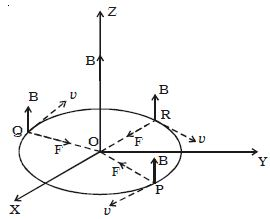Physics

# Experiment: Motion of a Charged Particle in a Uniform Magnetic Field

Experiment: Motion of a charged particle in a uniform magnetic field

Let consider a uniform magnetic field of induction B acting along the Z-axis. A particle of charge q and mass m moves in XY plane.

At a point P, the velocity of the particle is v. (Figure)

The magnetic lorentz force on the particle is F = q (v×B). Hence

F acts along PO perpendicular to the plane containing v and B.

Since the force acts perpendicular to its velocity, the force does not do any work. So, the magnitude of the velocity remains constant and only its direction changes. The force F acting towards the point O acts as the centripetal force and makes the particle to move along a circular path. At points Q and R, the particle experiences force along QO and RO respectively.Fig: Motion of a charged particle

Since v and B are at right angles to each other

F = Bqv sin 900 = Bqv

This magnetic lorentz force provides the necessary centripetal force.

Bqv = mv2 /r

r = mv/Bq … (1)

It is evident from this equation, that the radius of the circular path is proportional to (i) mass of the particle and (ii) velocity of the particle

From equation (1),

v/r = Bq/m

ω = Bq/m … (2)

This equation gives the angular frequency of the particle inside the magnetic field.

Period of rotation of the particle,

T = 2π/ω

T = 2πm/Bq … (3)

From equations (2) and (3), it is evident that the angular frequency and period of rotation of the particle in the magnetic field do not depend upon (i) the velocity of the particle and (ii) radius of the circular path.